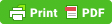# Lacking Natural Simplicity

Random musings on books, code, and tabletop games.

## Fun with Lisp: notf and cdrf

I actually use these in Emacs Lisp, for which you'd need a `(require 'cl))`, but the `define-modify-macro` is originally from Common Lisp.

These are trivial, but useful.

```(define-modify-macro notf (&rest args) not)
(setq x nil)
(notf x)
x ;;=> t
(notf x)
x ;;=> nil
(setq x ‘(a (b (c t))))
x ;;=> (a (b (c nil)))
x ;;=> (a (b (c t)))
(setq x [1 2 3 t 5])
(notf (aref x 3))
x ;;=> [1 2 3 nil 5]
(notf (aref x 3))
x ;;=> [1 2 3 t 5]

(define-modify-macro cdrf (&rest args) cdr)
(setq x ‘(:a :b :c :d 1 2 3))
(defun frob (keyword) ‘nothing-now)
(while (member (car x) ‘(:a :b :c :d))
(frob (car x))
(cdrf x))
x ;;=> (1 2 3)
```